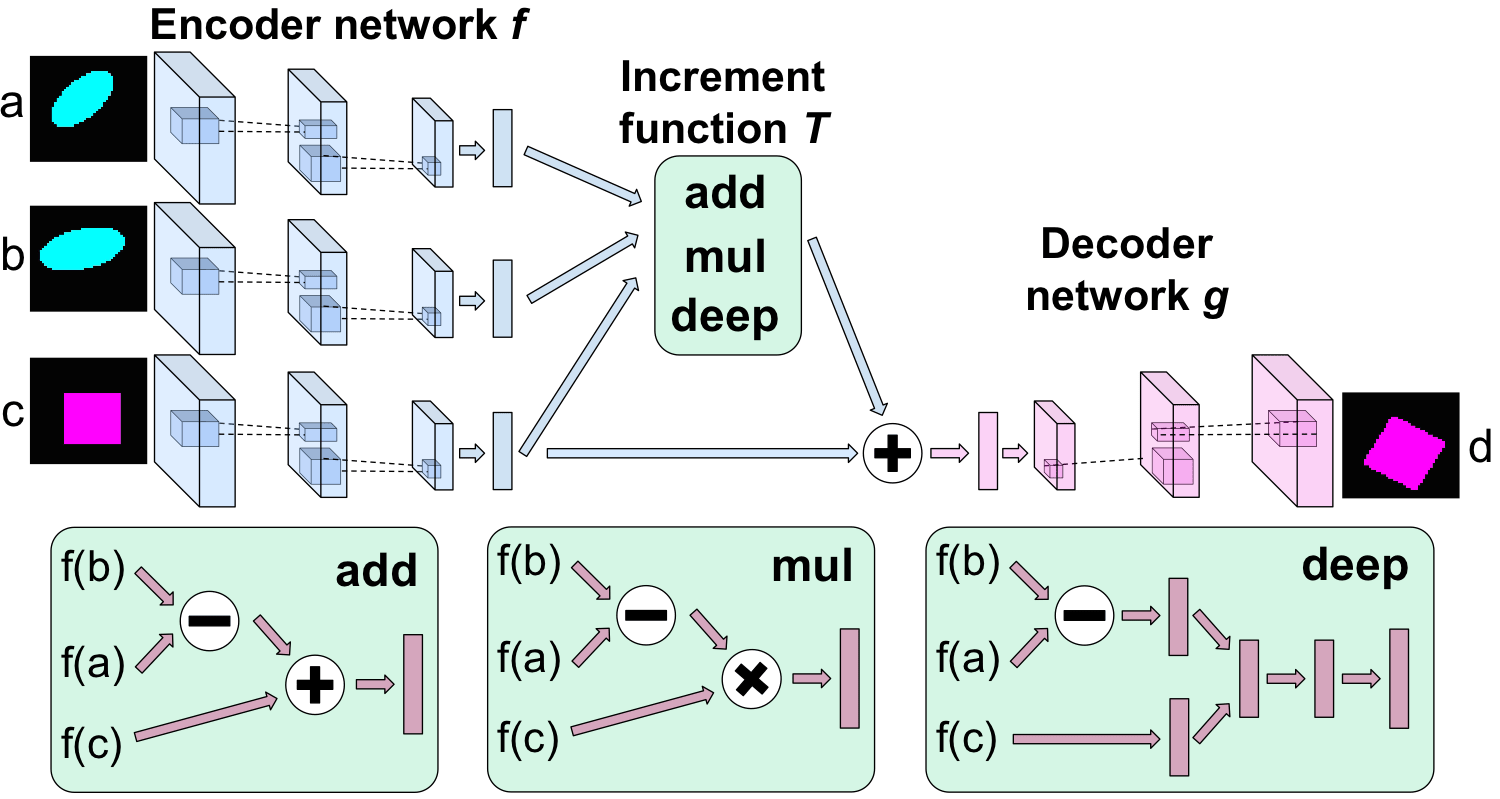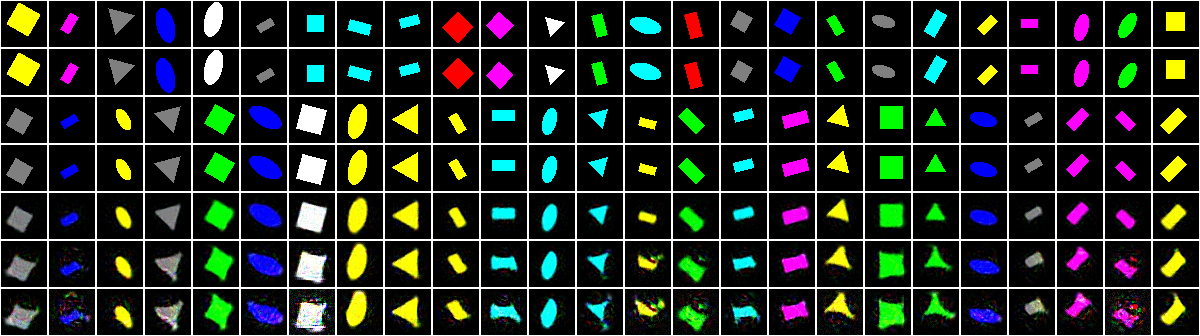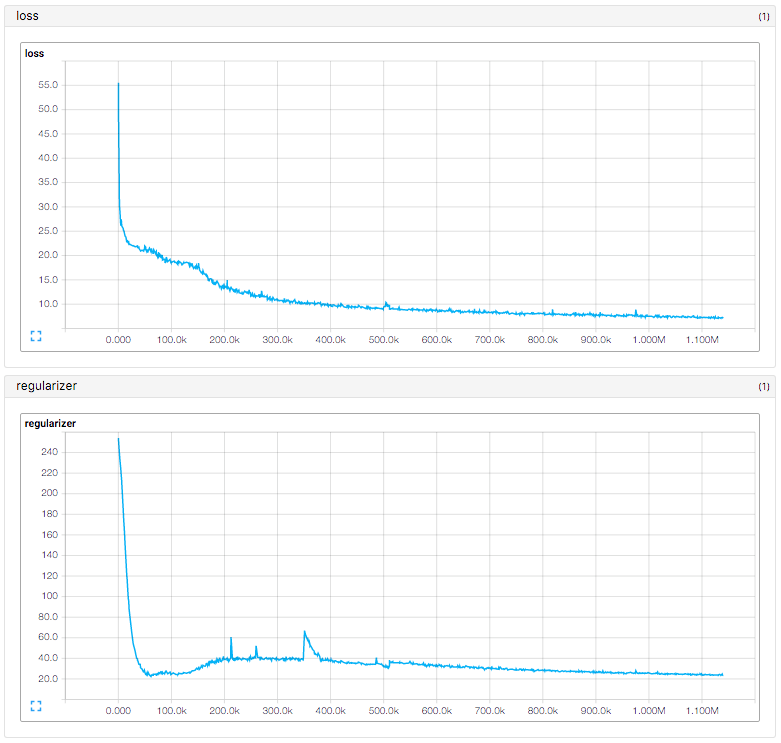Tensorflow implementation of Deep Visual Analogy-Making. The matlab code of the paper can be found here.This implementation contains a deep network trained end-to-end to perform visual analogy making with

1. Fully connected encoder & decoder networks
2. Analogy transformations by vector addition and deep networks (vector multiplication is not implemented)
3. Regularizer for manifold traversal transformations

This implementation conatins:

1. Analogy transformations of `shape` dataset
• with objective for multiple fully connected layers (L_deep)
• with manifold traversal transformations

## Usage

``````\$ ./download.sh
``````

To train a model with `shape` dataset:

``````\$ python main.py --dataset shape --is_train True
``````

To test a model with `shape` dataset:

``````\$ python main.py --dataset shape
``````

## Results

Result of analogy transformations of `shape` dataset with fully connected layers (L_deep) after 1 day of training.

From top to bottom for each : Reference, output, query, target, prediction, manifold prediction after 2 steps, and manifold prediction after 3 steps.(in progress)

## Training details## Author

Taehoon Kim / @carpedm20